Publication date: 03/23/2021

# Conditional Functions

You can include conditional expressions (called conditionals for short) in your formulas. These expressions let you build a sequence of clauses paired with result expressions. Constructing a sequence of clauses is the way you conditionally assign values to cells in a calculated column. Conditionals follow these rules:

When no clause is true, the Formula Editor evaluates the result expression that accompanies the else clause.

All result expressions in a conditional expression must evaluate to the same data type.

A missing term matches any data type.

By definition, expressions that evaluate as zero are false.

If an expression evaluates as missing, no clauses are executed and missing is returned. All other numeric expressions are true.

See Conditional Functions in the Scripting Guide and Conditional and Logical Functions in the JSL Syntax Reference for more information about syntax.

Use the insert and delete clause buttons on the Formula Editor panel to expand the expression. For maximum efficiency, list the most frequently evaluated clause and result pairs first in the sequence.

Note: Interpolate, Step, For, and While are most often used in conjunction with other commands to build a JSL script. You can use the Formula Editor to create and execute a script in that column, but this is not recommended because of dependencies and ambiguities that can result. Most often, scripts are stored as .jsl files, and can be saved with a data table as a table property. For more information about table properties, see Table Panel in Data Tables. For documentation of scripting commands, see the JSL Syntax Reference.

#### If

Shows a single If condition with a missing expression and a missing then clause. Highlight either expr or then clause and enter a value. For example, to calculate count as a percentage of total when total is not 0, enter the conditional expression (using columns called count and total) in Figure A.3.

Figure A.3 A Conditional Expression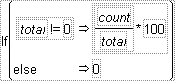To add a new condition to the If conditional, highlight then clause and click the insert button (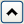) on the Formula Editor keypad. Initially, this changes the existing else condition to an expr clause. Click the insert button again to add an else clause. Highlighting then or else and repetitively clicking the insert button changes the else to expr or adds a new expr clause.

To delete a clause, select the then clause above it and press Delete or click the Delete button (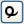) on the Formula Editor keypad.

By definition, expressions that evaluate as zero are false. If an expression evaluates as missing, no clauses are executed and missing is returned. All other numeric expressions are true.

#### Match

Compares an expression to a list of clauses and returns the value of the resulting expression for the first matching clause encountered. You provide the matching expression only once and then give a match value for each clause.

After you select Match in the Formula Editor, a list appears with two options:

Select Add Match Arguments from Data, and clauses that correspond to all of the levels in your data are added automatically. Alternatively, press Shift, select Conditional, and then select Match. In Figure A.4, the example on the left shows clauses that were added automatically.

Select Don’t Add so that you can add each clause individually. In Figure A.4, the example on the right shows an empty clause, which you fill with the missing expressions.

Figure A.4 Examples of Using the Match Function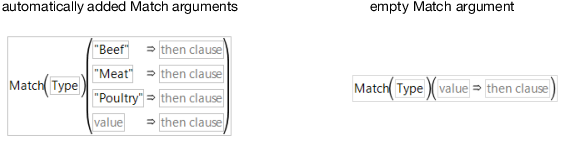In an automatically filled argument, you should highlight then clause, and then enter an expression. In an empty argument, you highlight either expr, value, or then clause, and then enter an expression. (Or, if you highlight an expression and click Match, the Formula Editor creates a new Match conditional, with the original highlighted expression as expr and nothing for the value and else clause.) Also, keep in mind that:

Match evaluates faster and uses less memory than an equivalent If because the variable is evaluated only once for each row in the data table. The If condition must evaluate the variable at each If clause for each row until a clause evaluates as true. See Comparison Functions, for a comparison of Match and If conditionals.

With If and Match, the Formula Editor searches down from the top of the sequence for the first true clause and evaluates the corresponding result expression. Subsequent true clauses are ignored.

In the following example, each value is assigned depending on the value of the age variable.

Figure A.5 An Example of Using the Match Function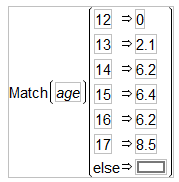Note: Match ignores trailing spaces and If does not.

Although Match returns missing for any missing values, you can also specifically match missing values.

#### Choose

Choose is a special case of Match in which the arguments of the condition are a sequence of integers starting at one. The value of clause replaces the match condition. An example of a Choose condition is shown in Figure A.6. With Choose, the Formula Editor goes directly to the correct choice clause and evaluates the result expression.

Figure A.6 Example of a Choose Condition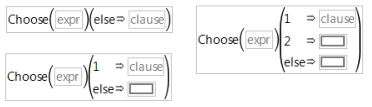When you highlight an expression and click Choose, the Formula Editor creates a new conditional expression with one clause. Use the insert () and delete () buttons on the keypad to add new clauses or remove unwanted clauses, as described previously for the If conditional.

Choose evaluates the Choose expression and goes immediately to the corresponding result expression to generate the returned value. With Choose, you provide a choosing expression that yields sequential integers starting at 1 only once, and then you give a choice for each integer in the sequence.

#### IfMax

Evaluates the first of each pair of arguments and returns the evaluation of the result expression (the second of each pair) associated with the maximum of the expressions. If more than one expression is the maximum, the first maximum is returned. If all expressions are missing and a final result is not specified, missing is returned. If all expressions are missing and a final result is specified, that final result is returned. The test expressions must evaluate to numeric values, but the result expressions can be anything.

#### IfMin

Evaluates the first of each pair of arguments and returns the evaluation of the result expression (the second of each pair) associated with the minimum of the expressions. If more than one expression is the minimum, the first minimum is returned. If all expressions are missing and a final result is not specified, missing is returned. If all expressions are missing and a final result is specified, that final result is returned. The test expressions must evaluate to numeric values, but the result expressions can be anything.

#### And &

Evaluates as 1 when both of its arguments are true. Otherwise, it evaluates as 0 (Figure A.9.) The formula in Figure A.7 labels Group 1 as drivers only if both comparisons are true.

Figure A.7 Creating an And Function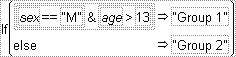#### Or |

Evaluates as 1 when either of its arguments is true. If both of its arguments are false, then the Or expression evaluates as 0 (Figure A.9.) The formula in Figure A.8 assigns males and all participants who are more than 13 years old to Group 1.

Figure A.8 Creating an Or Function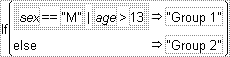The truth tables on the left in Figure A.9 illustrate the results of the And ( & ) and Or (| ) functions when both arguments have nonmissing values that evaluate to true or false. The table on the right illustrates the result when either the left or right expression (call them a and b) or both have missing values.

Figure A.9 Evaluations of And and Or Expressions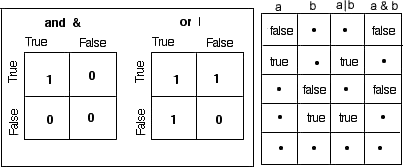#### Not !

Evaluates as 1 when its argument is false. Otherwise, Not evaluates as 0. When you apply the Not function, use parentheses where necessary to avoid ambiguity. For example, !(weight==64) can be either true or false (either 1 or 0), but (!weight)==64 is always false (0) because Not can return only 0 or 1. Expressions such as !(weight==64) can also be entered as weight != 64.

#### Interpolate

Linearly interpolates the y-value between two points, x1, y1 and x2, y2 that corresponds to the arguments that you give. You can insert additional pairs of x, y arguments with the Insert key. Interpolate finds the pair of x, y points that correspond to the x-value and completes the interpolation.

Figure A.10 Examples of Interpolate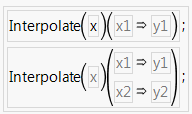#### Step

Step is like Interpolate except that it returns the y-value corresponding to the greatest x-value less than or equal to the x and y arguments. That is, it finds the corresponding y for a given x from a step function rather than a linear fit between points. Like Interpolate, you can have as many x and y argument pairs as you want.

Figure A.11 Examples of Step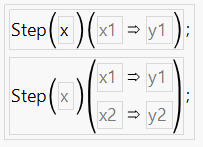#### For

Repeats the statements in the body argument as long as the while condition is true. The init and next control the iterations.

#### While

Repeatedly tests the expr condition and executes the body until expr is no longer true.

#### Break, Continue

Break stops execution of a loop completely and continues to the statement following the loop. Continue ends the current iteration of a loop and begins the loop at the next iteration.

Both are used in For, While, and For Each Row loops.

#### Stop

Immediately stops a script that is running.

#### Return

Returns an expression value from a user-defined function.

Want more information? Have questions? Get answers in the JMP User Community (community.jmp.com).